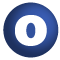# Position

#### object Position extends AnyRef

The object `Position` provides convenience methods to encode line and column number in one single integer. The encode line (column) numbers range from 0 to `LINE_MASK` (`COLUMN_MASK`), where 0 indicates that the line (column) is the undefined and 1 represents the first line (column). Line (Column) numbers greater than `LINE_MASK` (`COLUMN_MASK`) are replaced by `LINE_MASK` (`COLUMN_MASK`). Furthermore, if the encoded line number is `LINE_MASK`, the column number is always set to 0.

The following properties hold:

• the undefined position is 0: `encode(0,0) == 0`
• encodings are non-negative : `encode(line,column) >= 0`
• position order is preserved: `(line1 < line2) || (line1 == line2 && column1 < column2)`
implies
`encode(line1,column1) <= encode(line2,column2)`

1. AnyRef
2. Any

### Value Members

1. #### val COLUMN_BITS: Int

Number of bits used to encode the column number

Number of bits used to encode the column number

attributes: final

Mask to decode the column number

Mask to decode the column number

attributes: final
3. #### val FIRSTPOS: Int

The first position in a source file

The first position in a source file

attributes: final
4. #### val LINE_BITS: Int

Number of bits used to encode the line number

Number of bits used to encode the line number

attributes: final

Mask to decode the line number

Mask to decode the line number

attributes: final
6. #### val NOPOS: Int

The undefined position

The undefined position

attributes: final
7. #### def equals(arg0: Any): Boolean

This method is used to compare the receiver object (`this`) with the argument object (`arg0`) for equivalence

This method is used to compare the receiver object (`this`) with the argument object (`arg0`) for equivalence.

The default implementations of this method is an equivalence relation:

• It is reflexive: for any instance `x` of type `Any`, `x.equals(x)` should return `true`.
• It is symmetric: for any instances `x` and `y` of type `Any`, `x.equals(y)` should return `true` if and only if `y.equals(x)` returns `true`.
• It is transitive: for any instances `x`, `y`, and `z` of type `AnyRef` if `x.equals(y)` returns `true` and `y.equals(z)` returns `true`, then `x.equals(z)` should return `true`.

If you override this method, you should verify that your implementation remains an equivalence relation. Additionally, when overriding this method it is often necessary to override `hashCode` to ensure that objects that are "equal" (`o1.equals(o2)` returns `true`) hash to the same `Int` (`o1.hashCode.equals(o2.hashCode)`).

arg0

the object to compare against this object for equality.

returns

`true` if the receiver object is equivalent to the argument; `false` otherwise.

definition classes: AnyRef ⇐ Any
8. #### def hashCode(): Int

Returns a hash code value for the object

Returns a hash code value for the object.

The default hashing algorithm is platform dependent.

Note that it is allowed for two objects to have identical hash codes (`o1.hashCode.equals(o2.hashCode)`) yet not be equal (`o1.equals(o2)` returns `false`). A degenerate implementation could always return `0`. However, it is required that if two objects are equal (`o1.equals(o2)` returns `true`) that they have identical hash codes (`o1.hashCode.equals(o2.hashCode)`). Therefore, when overriding this method, be sure to verify that the behavior is consistent with the `equals` method.

definition classes: AnyRef ⇐ Any
9. #### def toString(pos: Int): String

Returns a string representation of the encoded position

Returns a string representation of the encoded position.

10. #### def toString(): String

Returns a string representation of the object

Returns a string representation of the object.

The default representation is platform dependent.

definition classes: AnyRef ⇐ Any